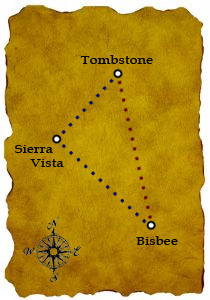SEARCH HOMEMath Central Quandaries & QueriesQuestion from cynthia, a student: Hi, If I have a question with a right triangle and it asks.... If ABC is say 400 miles. How much shorter will the miles be if I travel from BC? I don't exactly remember the question but, I would I solve a problem similiar to this one? Thanks for your help, CynthiaHi Cynthia.

Yes, this is a fairly common kind of question, so I think I know what you mean.

Here's an example I made up:Smiley Carson was living in Bisbee and had to get to Tombstone to work in his uncle's black smith shop. He was preparing for the long ride there with his wife Dot when she asked him if they could stop by Sierra Vista to see her mother, since it was kind of "on the way" anyhow.

It's 40 miles NW from Bisbee to Sierra Vista and 30 miles NE from there to Tombstone. How much further is it go from Bisbee to Tombstone by way of Sierra Vista than going directly?

Solution:
This is a right triangle, so you can use the Pythagorean Theorem to solve it. This says that h2 = a2 + b2 where h is the length of the hypotenuse (that's the side opposite the right angle) and the two legs a and b. So in this question, we have h2 = 402 + 302. That means h2 = 2500, so  h = 50 miles.

Thus it is 50 miles directly and 70 miles by way of Sierra Vista, so the difference is 20 miles.

Hope this example helps you, Cynthia.
Stephen La Rocque.Math Central is supported by the University of Regina and The Pacific Institute for the Mathematical Sciences.